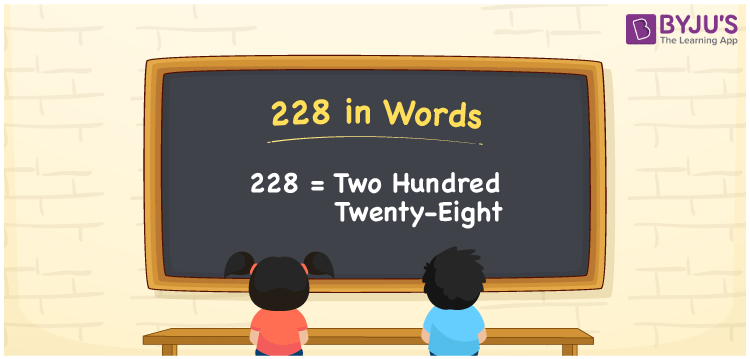# 228 in Words

We can write 228 in words as Two hundred twenty-eight. If you bought ice cream for Rs. 228, then you can say, “I bought an ice cream worth Two hundred twenty-eight rupees”. In this article, you will understand how to convert the cardinal number 228 into words with the help of a place value chart, along with some interesting facts about 228.

 228 in words Two hundred twenty-eight Two hundred twenty-eight in Numbers 228

## 228 in English Words

We generally express numbers in words using the English alphabet. Therefore, we can spell 228 in English words as “Two hundred twenty-eight”.## How to Write 228 in Words?

Let’s learn how to write the number 228 in words using a place value chart. The below table illustrates the place values for all three digits of 228.

 Hundreds Tens Ones 2 2 8

Here, ones = 8, tens = 2, hundreds = 2

These digits can be written according to their place values as follows.

2 × Hundred + 2 × Ten + 8 × One

= Two hundred + Twenty + Eight

= Two hundred twenty-eight

Therefore, 228 in words = Two hundred twenty-eight

228 is a natural number that precedes 229 and succeeds 227.

228 in words – Two hundred twenty-eight

Is 228 an odd number? – No

Is 228 an even number? – Yes

Is 228 a prime number? – No

Is 228 a composite number? – Yes

Is 228 a perfect square number? – No

Is 228 a perfect cube number? – No

## Frequently Asked Questions on 228 in Words

### Write the number 228 in word form.

The word form of the number 228 is Two hundred twenty-eight.

### How to write Rs. 228 in words on a cheque?

On a cheque, we can write 228 in words as “Two hundred twenty-eight rupees only”.

### Write the value of 228 + 500 in words.

228 + 500 = 728 Therefore, the value of 228 + 500, i.e., 728 in words is Seven hundred twenty-eight.